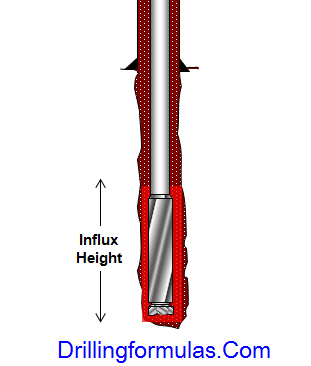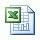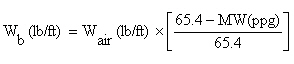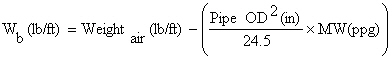## Formation Pressure from Kick Analysis

Once you shut in the well in and obtain shut in drill pipe pressure, you can estimate formation pressure by applying the hydrostatic pressure concept.

This following equation demonstrates you how to figure out formation pressure from the kick analysis.

Formation Pressure = SIDDP + (0.052 x Hole TVD x Current Mud Weight)

## Maximum influx height to equal the maximum allowable shut-in casing pressure

You know how the maximum allowable shut in casing pressure (MASICP) from the leak off test data and your current mud weight in the well. Moreover, you can use the MASICP to determine the maximum influx height that you can be able to control in the well control situation.The following formula shows how to determine the figure:

## Maximum formation pressure that can be controlled when we shut the well in

You know about kick tolerance factor from previous section and you can use it to determine the maximum formation pressure which can be controlled when the well is shut in.This equation below demonstrates you how to calculate it.

## Maximum surface pressure from kick tolerance factor

You have learned about the kick tolerance factor from the previous blog posting and this article will demonstrate you how to use the kick tolerance factor to determine the maximum surface pressure from it.

The formula is listed below;

Maximum surface pressure = kick tolerance factor x 0.052 x TVD of well

Where;

Maximum surface pressure in psi

kick tolerance factor in ppg

TVD of well in ft

Determine the maximum surface pressure with this following data:

Kick tolerance factor = 1.8 ppg

Hole depth = 10,000’MD/9500’TVD

Based on these data, you can calculate the maximum surface pressure like this;

Maximum surface pressure = 1.8 x 0.052 x 9500 = 889 psi

Please find the Excel sheet for this calculationMaximum surface pressure from kick tolerance factor

## Well Control Formulas Part 6

This part of well control formulas will demonstrate you more about some equations frequently used in snubbing operation. In Well Control Formulas Part 5, I show just only some snubbing formulas and this part will show the rest of them.

I wish you would enjoy reading my blog post.

Buoyed Weight of Open Ended Tubulars (Wb),lb

For this formula, you just use the buoyancy factor and multiply it with weight of pipe in the air.Buoyed Weight of Closed Ended Tubulars without fluid in the pipe (Wb),lb

This formula below is different from the 1st formula because it is used for the closed ended pipe. Weight in the air will be subtracted with buoyancy weight of pipe ran in hole.

Note: 24.5 is conversion factor to make the unit in gallon.